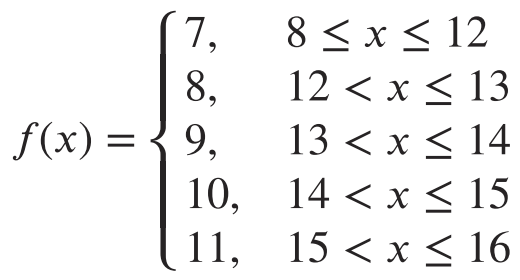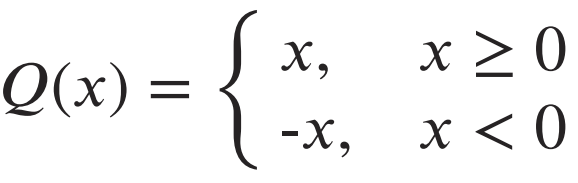# A.4.15 Practice Problems

## Problem 1

Noah's cousin is exactly 7 years younger than Noah. Let C represent Noah's cousin's age and N represent Noah's age. Ages are measured in years. a. Write a function that defines the cousin's age as a function of Noah's age. What are the input and output of this function? b. Write the inverse of the function you wrote. What are the input and output of this inverse function?

## Problem 2

Noah's cousin is exactly 7 years younger than Noah. Let M represent Noah's cousin's age in months and N represent Noah's age in years. a. If Noah is 15 years old, how old is his cousin, in months? b. When Noah's cousin is 132 months old, how old is Noah, in years? c. Write a function that gives the age of Noah's cousin in months, as a function of Noah's age in years. d. Write the inverse of the function you wrote. What are the input and the output of this inverse function?

## Problem 3

Each equation represents a function. For each, find the inverse function. a. c=w+3 b. y=x-2 c. y=5x d. w=d/7

## Problem 4

Sketch a graph to represent each quantity described as a function of time. Be sure to label the vertical axis.

## Problem 5

The number of years, y, is a function of the number of months, m. The number of months, m, is also a function of the number of years, y. a. Write two equations, one to represent each function. b. Explain why the two functions are inverses.

## Problem 6

Lin charges \$5.50 per hour to babysit. The amount of money earned, in dollars, is a function of the number of hours that she babysits. Which of the following inputs is impossible for this function?

Check all that apply

## Problem 7

The instructions for cooking a steak with a pressure cooker can be represented with this set of rules, where x represents the weight of a steak in ounces and f(x) the cooking time in minutes.a. Describe the instructions in words so that they can be followed by someone using the pressure cooker.

## Problem 8

The absolute value function Q(x)=|x| gives the distance from 0 of the point x on the number line. Q can also be defined using piecewise notation:Determine if each point is on the graph of Q. For each point that you believe is not on the graph of Q, change the output coordinate so that the point is on the graph of Q. a. (-3,3) b. (0,0) c. (-5,-5) d. (-72,72) e. (4/5,-4/5)